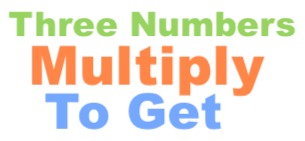What three numbers multiply to get 120?What three numbers multiply to get 120? In other words, you want to know all the combinations of 3 numbers that can be multiplied to get 120. You want to know what all the question marks in the equation below can be in order for the equation to be true:

? × ? × ? = 120

First, note that all numbers must be 120 or less, which means that there are 120 × 120 × 120 possible solutions. Thus, to get the answer to "What three numbers multiply to get 120?" we looked at 1,728,000 different combinations to see which ones equal 120.

When we looked at all those combinations, we found 90 different combinations of 3 numbers that equal 120 when multiplied together. Here are all 3 number combinations that you can multiply to get 120:

120 × 1 × 1 = 120
60 × 2 × 1 = 120
60 × 1 × 2 = 120
40 × 3 × 1 = 120
40 × 1 × 3 = 120
30 × 4 × 1 = 120
30 × 2 × 2 = 120
30 × 1 × 4 = 120
24 × 5 × 1 = 120
24 × 1 × 5 = 120
20 × 6 × 1 = 120
20 × 3 × 2 = 120
20 × 2 × 3 = 120
20 × 1 × 6 = 120
15 × 8 × 1 = 120
15 × 4 × 2 = 120
15 × 2 × 4 = 120
15 × 1 × 8 = 120
12 × 10 × 1 = 120
12 × 5 × 2 = 120
12 × 2 × 5 = 120
12 × 1 × 10 = 120
10 × 12 × 1 = 120
10 × 6 × 2 = 120
10 × 4 × 3 = 120
10 × 3 × 4 = 120
10 × 2 × 6 = 120
10 × 1 × 12 = 120
8 × 15 × 1 = 120
8 × 5 × 3 = 120
8 × 3 × 5 = 120
8 × 1 × 15 = 120
6 × 20 × 1 = 120
6 × 10 × 2 = 120
6 × 5 × 4 = 120
6 × 4 × 5 = 120
6 × 2 × 10 = 120
6 × 1 × 20 = 120
5 × 24 × 1 = 120
5 × 12 × 2 = 120
5 × 8 × 3 = 120
5 × 6 × 4 = 120
5 × 4 × 6 = 120
5 × 3 × 8 = 120
5 × 2 × 12 = 120
5 × 1 × 24 = 120
4 × 30 × 1 = 120
4 × 15 × 2 = 120
4 × 10 × 3 = 120
4 × 6 × 5 = 120
4 × 5 × 6 = 120
4 × 3 × 10 = 120
4 × 2 × 15 = 120
4 × 1 × 30 = 120
3 × 40 × 1 = 120
3 × 20 × 2 = 120
3 × 10 × 4 = 120
3 × 8 × 5 = 120
3 × 5 × 8 = 120
3 × 4 × 10 = 120
3 × 2 × 20 = 120
3 × 1 × 40 = 120
2 × 60 × 1 = 120
2 × 30 × 2 = 120
2 × 20 × 3 = 120
2 × 15 × 4 = 120
2 × 12 × 5 = 120
2 × 10 × 6 = 120
2 × 6 × 10 = 120
2 × 5 × 12 = 120
2 × 4 × 15 = 120
2 × 3 × 20 = 120
2 × 2 × 30 = 120
2 × 1 × 60 = 120
1 × 120 × 1 = 120
1 × 60 × 2 = 120
1 × 40 × 3 = 120
1 × 30 × 4 = 120
1 × 24 × 5 = 120
1 × 20 × 6 = 120
1 × 15 × 8 = 120
1 × 12 × 10 = 120
1 × 10 × 12 = 120
1 × 8 × 15 = 120
1 × 6 × 20 = 120
1 × 5 × 24 = 120
1 × 4 × 30 = 120
1 × 3 × 40 = 120
1 × 2 × 60 = 120
1 × 1 × 120 = 120

Three Numbers Multiply to Get Calculator
Do you need a different combination of three numbers that when multiplied equal a number other than 120? No problem! Please enter your number here.

What three numbers multiply to get 121?
Here is the next problem on our list that we have explained and answered for your convenience.